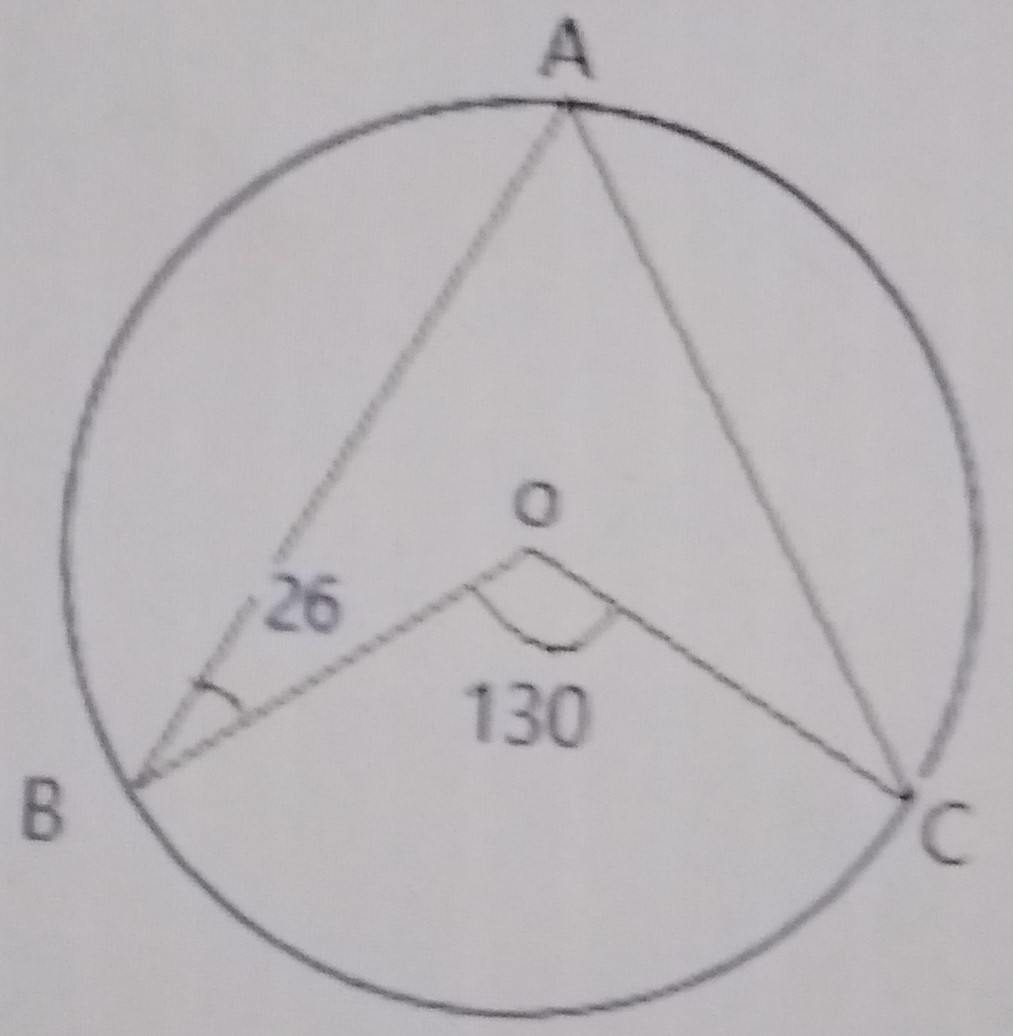### Mathematics Past Questions

4066

Find the value of x and y in the simultaneous equation: 3x + y = 21; xy = 30

• A. x = 3 or 7, y = 12 or 8
• B. x = 6 or 1, y = 11 or 5
• C. x = 2 or 5, y = 15 or 6
• D. x = 1 or 5, y = 10 or 7
4067

Points X and Y are 20km North and 9km East of point O, respectively. What is the bearing of Y from X? Correct to the nearest degree.

• A. 24°
• B. 56°
• C. 127°
• D. 156°
4068

If $$P = (\frac{Q(R - T)}{15})^{\frac{1}{3}}$$, make T the subject of the formula.

• A. $$T = \frac{15R - Q}{P^3}$$
• B. $$T = R - \frac{15P^3}{Q}$$
• C. $$T = \frac{R - 15P^3}{Q}$$
• D. $$T = \frac{R + P^3}{15Q}$$
4069In the diagram above, O is the centre of the circle ABC, < ABO = 26° and < BOC = 130°. Calculate < AOC.

• A. 26°
• B. 13°
• C. 80°
• D. 102°
4070

Each of the interior angles of a regular polygon is 140°. Calculate the sum of all the interior angles of the polygon.

• A. 1080°
• B. 1260°
• C. 2160°
• D. 1800°